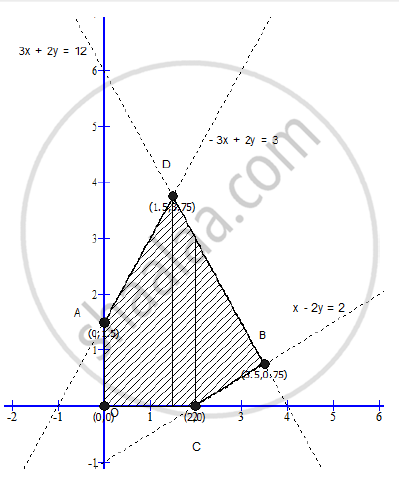HSC Arts 12th Board ExamMaharashtra State Board
Share

# Minimum and Maximum Z = 5x + 2y Subject to the Following Constraints: - HSC Arts 12th Board Exam - Mathematics and Statistics

ConceptGraphical Method of Solving Linear Programming Problems

#### Question

Minimum and maximum z = 5x + 2y subject to the following constraints:

x-2y ≤ 2

3x+2y ≤ 12

-3x+2y ≤ 3

x ≥ 0,y ≥ 0

#### Solution

x-2y ≤ 2

3x+2y ≤ 12

-3x+2y ≤ 3

x ≥ 0,y ≥ 0

Converting the inequations into equations, we obtain the lines

x-2y = 2 .....(i)

3x+2y = 12......(ii)

-3x+2y = 3.......(iii)

x = 0,y =0From the graph, we get the corner points as
A(0, 5), B(3.5, 0.75), C(2, 0), D(1.5, 3.75), O(0, 0)

The values of the objective function are:

 Point (x, y) Values of the objective functionZ = 5x + 2y A(0, 5) 5 × 0 + 2 × 5 = 10 B(3.5, 0.75) 5 × 3.5 + 2 × 0.75 = 19 (Maximum) C(2, 0) 5 × 2 + 2 × 0= 10 D(1.5, 3.75) 5 × 1.5 + 2 × 3.75 = 15 O(0, 0) 5 × 0 + 2 × 0 = 0 (Minimum)

The maximum value of Z is 19 and its minimum value is 0.

Is there an error in this question or solution?

#### Video TutorialsVIEW ALL 

Solution Minimum and Maximum Z = 5x + 2y Subject to the Following Constraints: Concept: Graphical Method of Solving Linear Programming Problems.
S